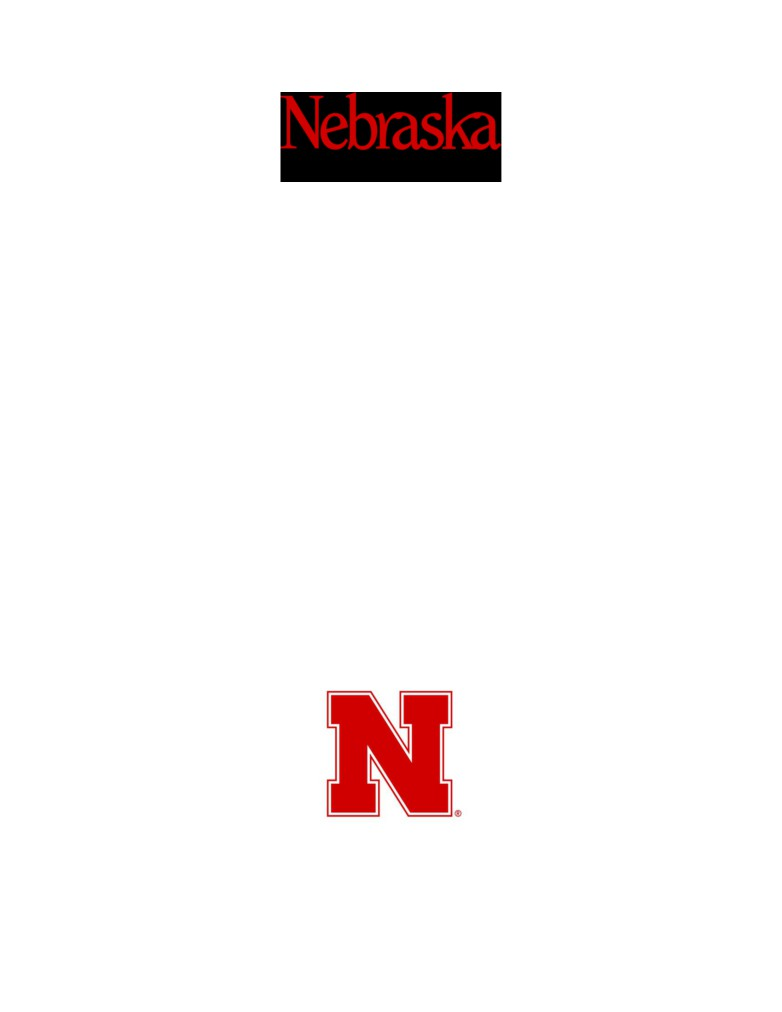ECEN-4330
Microprocessor Systems Design
Final Lab Report
Daniel Faronbi
Spring 2019
Department of Electrical
&
Computer Engineering
(Omaha Campus)Daniel Faronbi
2
1 Summary
2 Objective
3 Introduction
4 Hardware Discussion
5 Software Discussion
6 Problem Discussion
7 Conclusion
8 References
9 AppendixDaniel Faronbi
3
1 Summary
Microprocessors are all around us. They are used to power almost every electronic device in
our modern world. As such, learning how to design a microprocessor system is a must for every
computer engineer. In this class, we were tasked with creating a microprocessor system that
used an 8051 microcontroller as its core. The 8051 microcontrollers can be used for a variety of
tasks. In this case, we interfaced the microcontroller with ROM, RAM and multiple IO devices. The
hardware was prototyped on a breadboard then finalized with a PCB. We then programmed a simple
operating system for our minicomputer.
2 Objective
The objective of this lab was to give students real world experience with building a
microprocessor system. Construction of electronic systems with many different components
often takes a large of amount of time and effort. This is because of the work and the effort that
goes into debugging hardware problems. As such, this project also aims to teach students how
to debug hardware properly. We used various tools to debug our hardware such as logic
analyzers, voltmeters, etc. This helps students formulate organized steps to run through when
hardware does not work as expected.
3 Introduction
The purpose of this project was to give students in depth experience in designing a
microprocessor system. we needed to interface the 8051 with ROM, RAM and Multiple input
and output devices. We used two 32K rom chips, two 32K ram chips, a seven-segment display,
real time clock, analog to digital converter, temperature sensor, LCD, and keypad. Students
were asked to design every single aspect of the system. First, we had to design the schematic.
Next, we had to construct the hardware for the system. After that, we had to design and order
a printed circuit board for the system. Finally, we constructed the board on a printed circuit
board and programmed the software for the system.
4 Hardware Discussion
The hardware design took the most work when constructing this project. I have decided to
discuss the design of each element individually. The rest of this section will contain hardware
discussions of the MCU, decoding, ROM/RAM, LCD, keypad, seven-segment display, real-time
After I have finished my discussion of the hardware design, I will discuss the processes that
went into the design of the printed circuit board. This will include the schematic design, board
design, and the soldering of the components unto the board.Daniel Faronbi
4
Hardware design
MCU
The MCU was connected to a variety of lines. To get the device powered on, the RST pin was
connected to a push switch. This tied the line to VCC whenever a reset was needed.
The XTAL2 pin was connected to the crystal oscillator. This provided a clock frequency for the
microcontroller.
The ~EA/VPP pin was tied to ground to ensure that the microcontroller was reading from
external memory.
Port0 was used for both data and lower address lines (A0-A7). Using the ALE output from the
MCU and a data latch circuit, the address and the data lines could be separated.
Port1 was connected to the keypad.
Port2 was used for the higher address lines (A8-A15) and was mostly connected to ROM and
RAM.
Port3 was used for single pin control lines. The IO/~M pin was set to come out of P3.4. This pin
was used to differentiate between RAM and IO memory spaces. The CD pin used for the LCD
came out of P3.3. Also, the ~WR and ~RD pins came out of P3.6 and P3.7 respectively.
Decoding
To chip select ROM, RAM, and IO devices. Pal decoding was used. This took in a15, a14, PSEN,
IO/~M, and ~WR. As input. This had chip select for all devices as output. A layout of the decoder
is available in Figure 5 of the appendix. The logic used to implement this decoding is available
below.
romLow
= ( a15 );
romHigh
= ( !a15 );
ramLow
= !( !a15 & psen & !io );Daniel Faronbi
5
ramHigh
= !( a15 & psen & !io );
lcd
= !( !a15 & !a14 & psen & io );
sevenSeg
= !( !a15 & a14 & psen & io & !wr );
rtc
= !( a15 & !a14 & psen & io );
temperature
= !( a15 & a14 & psen & io );
ROM/RAM
ROM and RAM had very similar connections. They were both connected to the address and
data lines and to their respective chip selects. However, the R M chips’ write enable were
connected to the ~WR pin while the ROM chips’ write enable were tied to ground.
LCD
The LCD display was tied to the data lines, chip select, ~WR, and CD control lines to pick
between the command and data register of the devices. ~RD was connected to VCC, since data
was never read from the LCD.
The Keypad was connected to port1 of the MCU.
Seven-Segment Display
The Seven-Segment Display was connected to a data latch before being interfaced with the
data bus. The chip select of the display was the output enable of the data latch.
Realtime Clock
Both the realtime clock’s data and address lines were connected to port 0 of the MCU. This is
because the ALE of the MCU was also connected to this device. The clock uses this to
differentiate between addresses and data lines.
The RTC was also connected to its chip select and the ~RD and ~WR pins.
Analog to Digital Converter
to digital bits.
This device was also connected to the data bus, chip select, ~RD, and voltage reference lines.Daniel Faronbi
6
Printed Circuit Board Design
To construct the printed circuit board, Eagle Cad was used. My original plan was to use OrCad.
However, the schematic and board design were much easier using Eagle Cad. Before designing
the schematic or the board, I had to find schematic symbols and footprints for all the
components used on my board. Specific websites used for finding this information is available
in the appendix section of this report
Schematic Design
The schematic was designed using Eagle Cad’s schematic editor. Different sections of the circuit
were separated to make the design easy to understand at first glance. Busses were used for
data lines and addresses to group wires of similar types. Port outputs were added, so that
debugging would be much easier. This also gives me the possibility of expanding the system in
the future. Figures 1-4 show the schematic design of this project.
Board Design
In eagle, the board and schematic files are linked. If a component is added to the schematic file,
it must be added to the board file as well. I placed all the components in an order that I felt
made sense. Next, I drew 10 mil traces of each of the necessary wires. Once all the traces were
done, I generated the Gerber files and ordered my circuit board from JLCPCB. Figure 6 shows
the board design of this project.
Board Construction
Once the board was delivered. I began soldering on all the components. At first, I only soldered
on the LCD, Rom Low, MCU, Decoding, address latch, and power circuitry. This was to test if the
device was working properly. I powered on the device, and nothing was displaying on the LCD.
After hours of looking for the problem, I realized that I tied The LCD RD pin to ground. I used a
nice to scratch out the trace from the PCB and soldered the RD pin to VCC. After this, the device
worked. I then soldered on the rest of the components.Daniel Faronbi
7
5 Software Discussion
All code is available in the appendix section of this report.
The Software of this project was meant to show that every component of the microprocessor
system was working correctly. The LCD and seven segment displays were used to give the User
visual feedback, the matrix keypad was used to input values to the microcontroller. There are 5
main functions I will discuss within my C program: Dump, Move, Edit, Find, and ioTest. Once the
devices boots up, the user is presented with a menu to choose one of the 5 different modes. All
the code was written in C, and the small device c compiler (sdcc) was used to compile the code.
Figure 8 shows a flowchart of the project’s user interface.
Dump
The dump function displays data on the screen. The data is shown based on the block size and
block type specified by the user. The program will show different sizes of data based on how
much was specified for each variable. A byte, word, or double word block type could be
selected with a block size of up to 16k. Once the block type, block size, and starting address
were specified. The dump function displayed the contents of external memory at the specified
address. The user has the option to increment or decrement the page number. Each page
displays one byte, word, or double word depending on what the user specifies.
Pointers with the __xdata prefix were used to read memory from external data and IO memory.
Move
The move function moved a block of data specified from one address to another address. The
user selects the block size and the starting address for the source and destination. Again,
pointers were used to read and write the data.Daniel Faronbi
8
Edit
The edit function takes an address specified by the user and prompts the user to enter in a new
value. The user then has the option of editing the next address or exiting the function.
Find
The find function ask the user for a specific 8-bit value, a starting address, and a block size. The
function will then search the block size at the starting address for the value specified. The
function will then return the addresses for all the places the value was found.
ioTest
This function test the IO devices connected to the 8051. The seven segment display counts from
1 to F (in hexadecimal) and the ADC and RTC display their values on the LCD.
6 Problem Discussion
I had a variety of problems when performing this lab. Many of them were small issues that took
me a while to figure out. I have decided to list my main four problems here, as talking about all
the issues I had would take too much time. Here are my main issues: Not reading seven
segment schematics before using, declaring ports as inputs/outputs, output enable on ram, and
missing bottom traces on PCB.
Not reading seven segment schematic before using
When first using the seven-segment display, I assumed that it must be connected to VCC and
ground. This was a huge mistake. Obviously, my breadboard was short circuited as a result. I
spent hours trying to figure out what wiring problem caused the issue before realizing the
simplicity of my error.
Declaring ports as inputs/outputs
When taught to program in 8051 assembly, I was told that we must write a 1 to a port to make
it an input and a 0 to a port to make it an output. This was not true. While testing my seven-
segment display, I realized that the IO/m pin was not changing. This is because wrote a zero to
it to be an output. Later I realized that this was not necessary, and my code worked as
expected.
Output enable on ram.
The first time I implemented my rom. I tied the output enable to ground. This was a mistake.
The data from the memory was constantly being output and caused bus collisions. I later
realized that I needed to connect this pin the ~RD pin of my MCU.Daniel Faronbi
9
Missing bottom traces on PCB
When ordering my first PCB, I accidentally uploaded my gerber files incorrectly. The
bottom
copper layer was not included. This resulted in a circuit board that did not have traces on
the
bottom. As such, this board was useless, and I had to order a new one.
PCB Issues
When my second PCB arrived, it had two issues. The RD pin of the LCD was tied to ground
and the LE and OE pins of the seven segment display data latch were not connected to
anything. To fix the LCD issue, I scratched off the trace connected to the RD pin on the PCB
and soldered a wire from the RD pin to VCC.
To fix the seven-segment latch issue, I simply solder two wires. One from the LE pin of the
latch to VCC. And one from the OE pin of the latch to the decoder’s seven-segment output.
7 Conclusion
After completing this project, I now am very familiar with the processes of designing a
microprocessor system. Interfacing with ROM, RAM and Multiple input and output devices is no
longer a hassle. After designing the system, schematic, and breadboard, I feel confident in my
ability to build a microprocessor system. This project took about over 200 hours to complete
and over \$350 dollars in expenses. (including parts and PCBs ordered). The list of parts used is
available in figure 7 of the appendix.
Figures 9-15 show pictures of the project. Click here for a link to a video demonstration of the
device.Daniel Faronbi
10
8 References
8051 Pin Out
ROM Data Sheet
RAM Data Sheet
Crystal Oscillator Data Sheet
LCD Data Sheet
Temperature Sensor Data Sheet
Analog to Digital Converter Data Sheet
Real Time Clock Datasheet
Site Used for Eagle Footprints
PCB Manufacturer
Small Device C Comppiler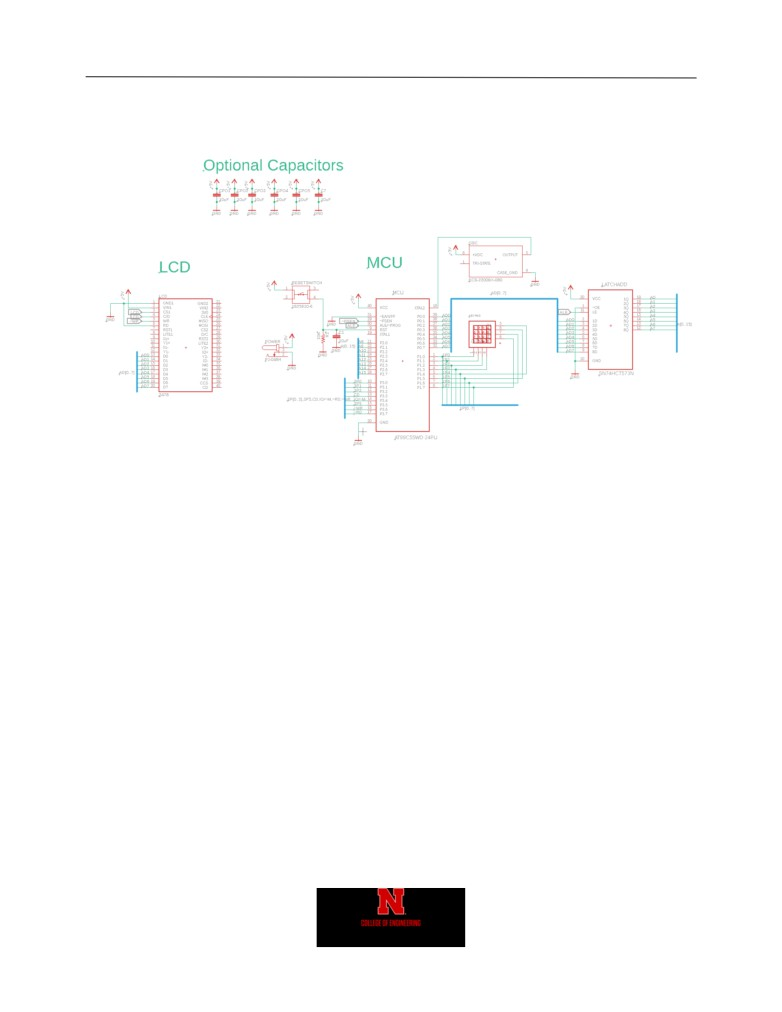Daniel Faronbi
11
9 Appendix
Figure 1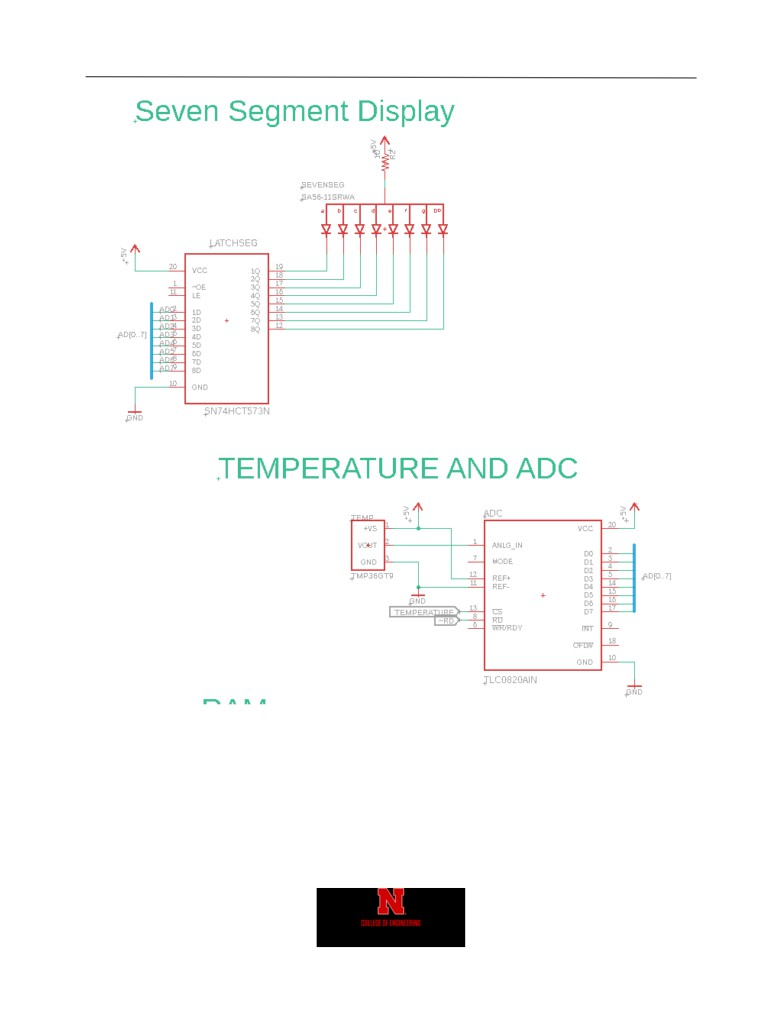Daniel Faronbi
12
Figure 2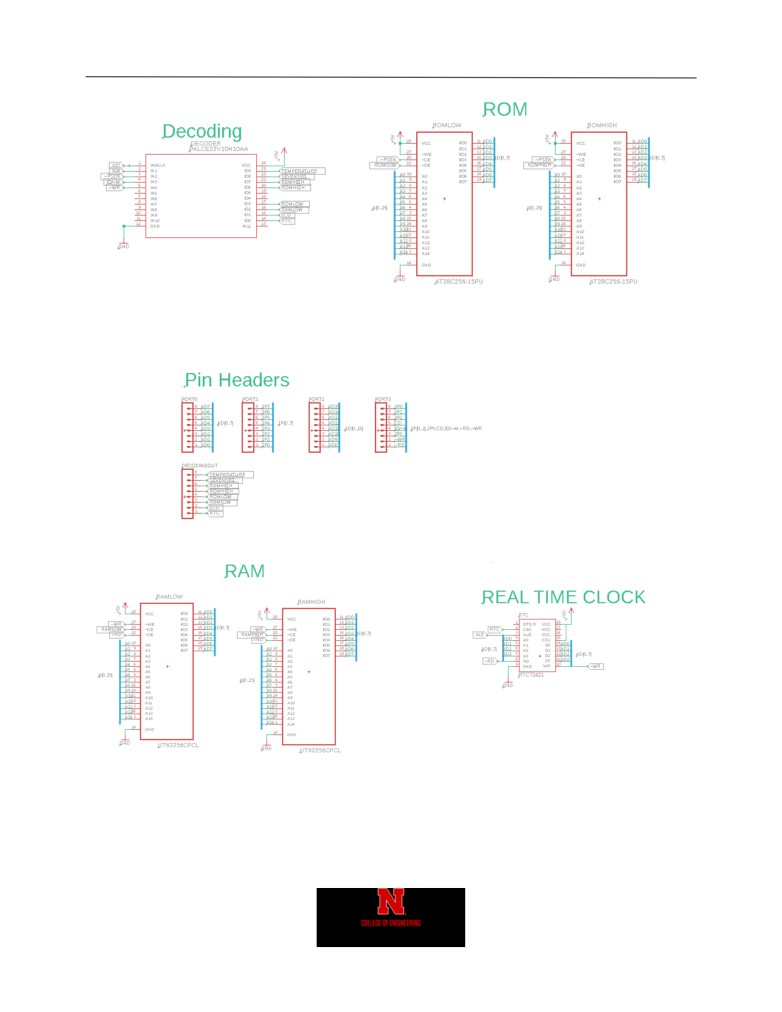Daniel Faronbi
13
Figure 3
Figure 4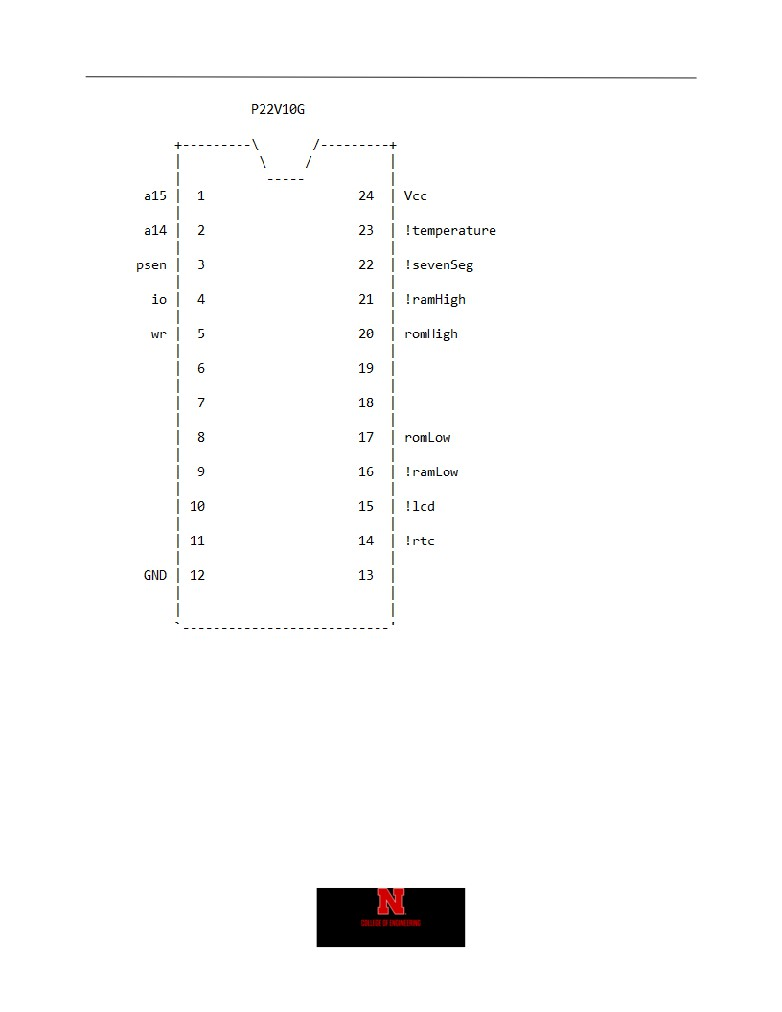Daniel Faronbi
14
Figure 5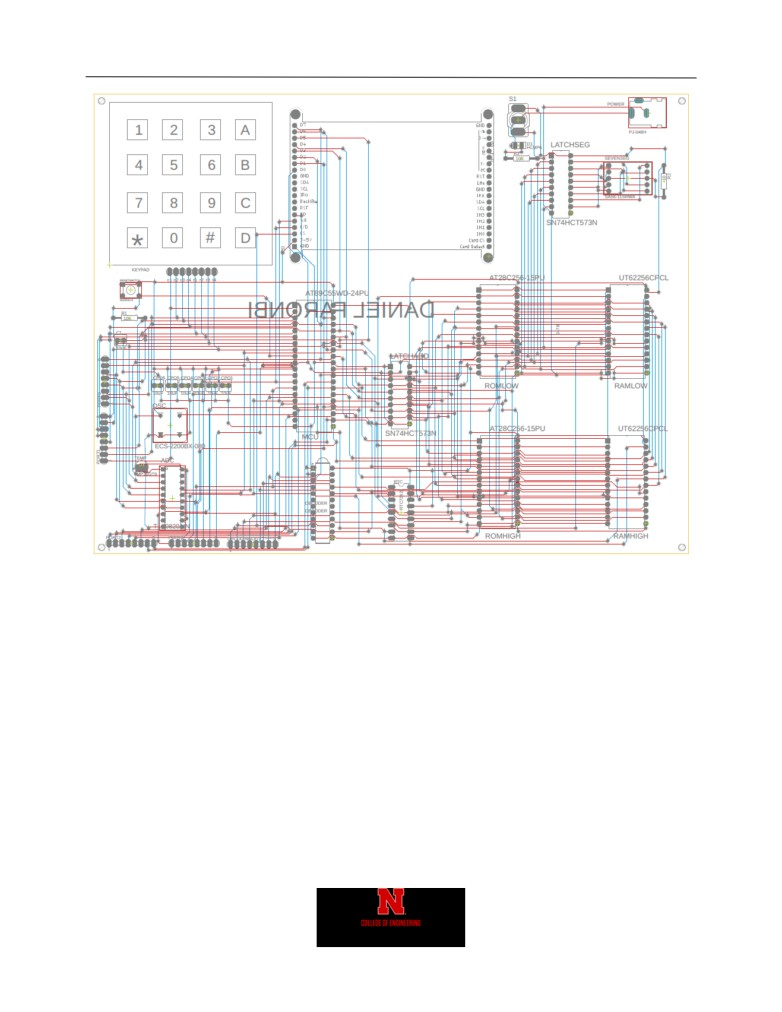Daniel Faronbi
15
Figure 6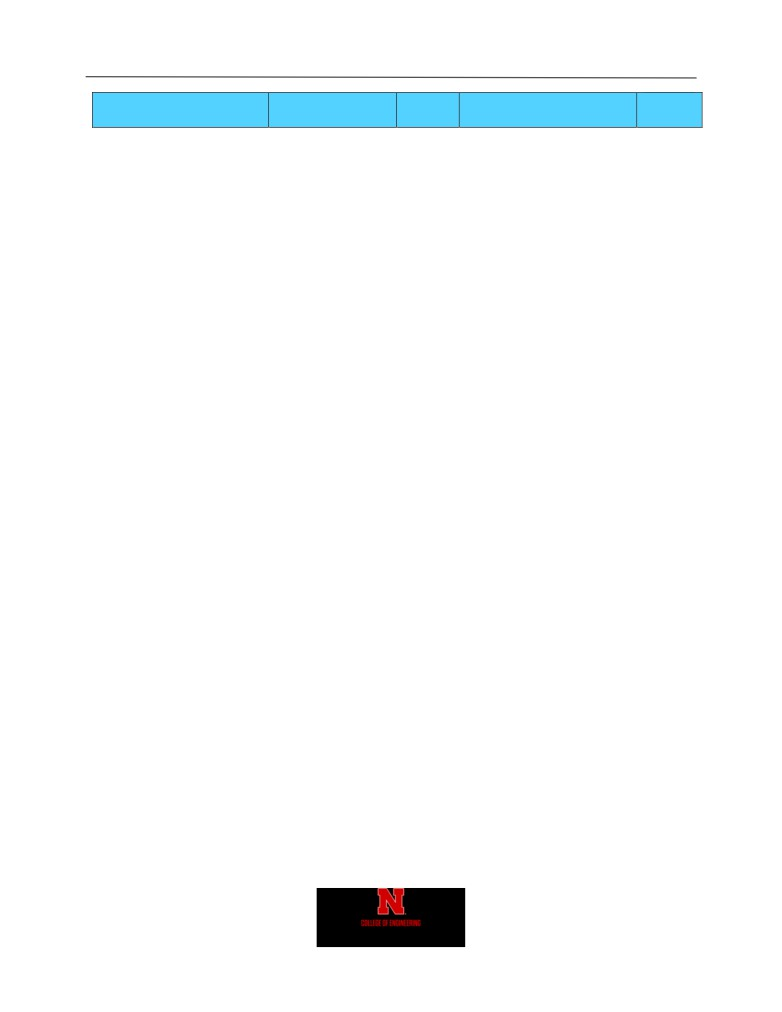Daniel Faronbi
16
Quantit
Part Name
Part Specification
Vendor
Available Part Number
y
Socket - 0.6"
40-pin processor
DigiKey
ED3048-5-ND
1
Socket - 0.6"
28-pin RAM
DigiKey
3M5469-ND
2
Socket - 0.3"
24-pin GAL
DigiKey
3M5466-ND
2
Socket - 0.3"
DigiKey
3M5465-ND
6
Socket - 0.3"
18-pin RTC
DigiKey
AE9995-ND
1
ZIF Socket
28-pin ROM
DigiKey
A302-ND
2
Microprocessor
AT89C55HWD
DigiKey
AT89C55WD-24PU-ND
1
TLC0820ACN
DigiKey
296-2849-5-ND
1
Temperature Sensor
TMP36GT9Z
DigiKey
TMP36GT9Z-ND
1
8-bit Latch/Demultiplexer
74HCT573
DigiKey
296-1621-5-ND
5
7-Segment LED (Common A)
SA56-11SRWA
DigiKey
754-1460-5-ND
1
RTC
RTC-72421
DigiKey
SER3231-ND
1
Crystal (Oscillator)
12 MHz
DigiKey
XC269-ND
1
Toggle switch
SPDT
DigiKey
EG2355-ND
2
SPST Push-Button Switch
Reset Signal
DigiKey
450-1650-ND
1
Screw potentiometer
10 K
DigiKey
3362P-103LF-ND
1
Fuse
2.5 A
DigiKey
F2400-ND
4
Fuse holder
Single 2-Pin Clip
DigiKey
F4189-ND
2
DigiKey
S7041-ND
1
DigiKey
S1011EC-40-ND
1
LCD mount
DigiKey
S7014-ND
1
Sensors
DigiKey
S7022-ND
1
Capacitor
Electrolytic 10uF
DigiKey
493-10487-1-ND
2
Capacitor
Ceramic 0.1 uF
DigiKey
BC1160CT-ND
20
Resistor
100 ohm
DigiKey
CF14JT100RCT-ND
10
Resistor
330 ohm
DigiKey
CF14JT330RCT-ND
10
Resistor
220 ohm
DigiKey
CF14JT220RCT-ND
10
Resistor
1K ohm
DigiKey
CF14JT1K00CT-ND
20
Resistor
10K ohm
DigiKey
CF14JT10K0CT-ND
10
RAM 32kx8
62256-lp70
Jameco
82472
2
EEPROM 32kx8
AT28C256-15PU
DigiKey
AT28C256-15PU-ND
2
GAL
GAL22V10D
Jameco
39167
3
Anti-Static Foam
DigiKey
16-1231-ND
1
C566C-RFS-CT0W0BB2CT-
Red LED
Power indicator
DigiKey
ND
1
Diode
1N4001
Jameco
35975
2
DC power jack
2.1mm
Jameco
101179
1
5V DC
Jameco
252736
1
Figure 7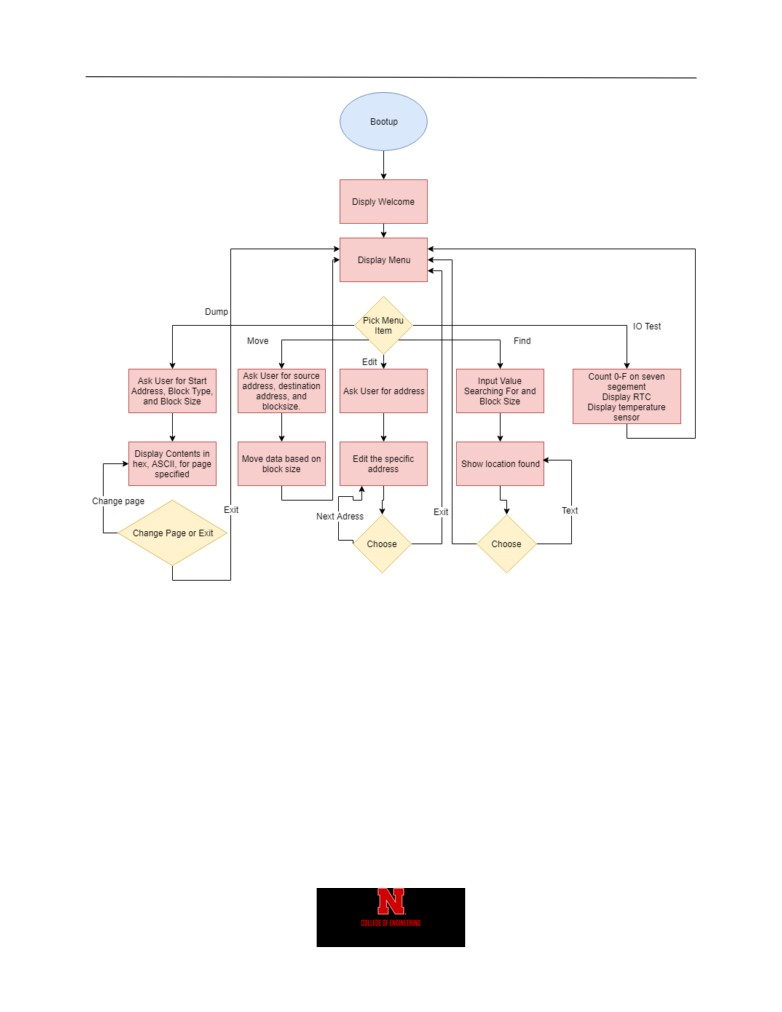Daniel Faronbi
17
Figure 8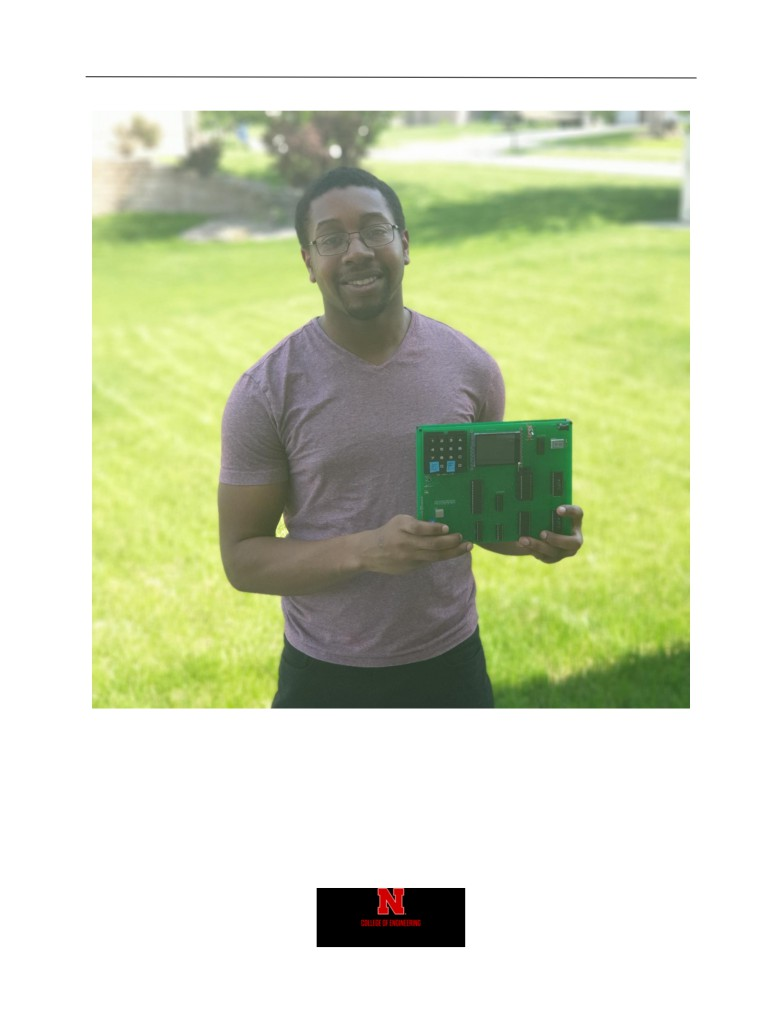Daniel Faronbi
18
Figure 9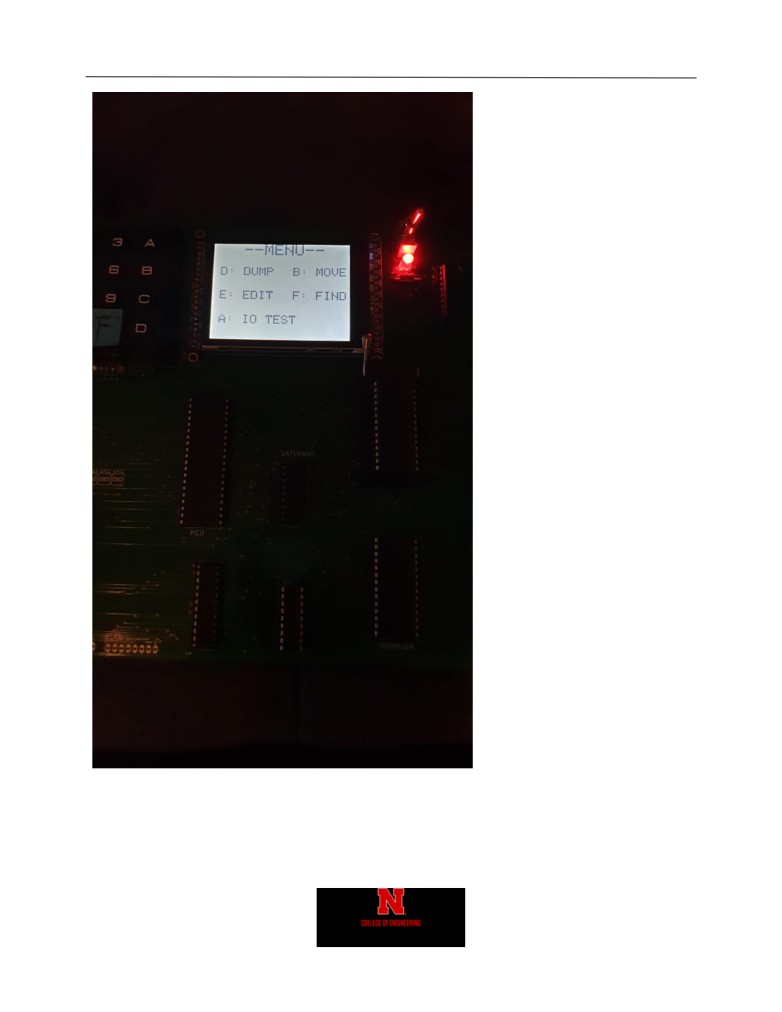Daniel Faronbi
19
Figure 10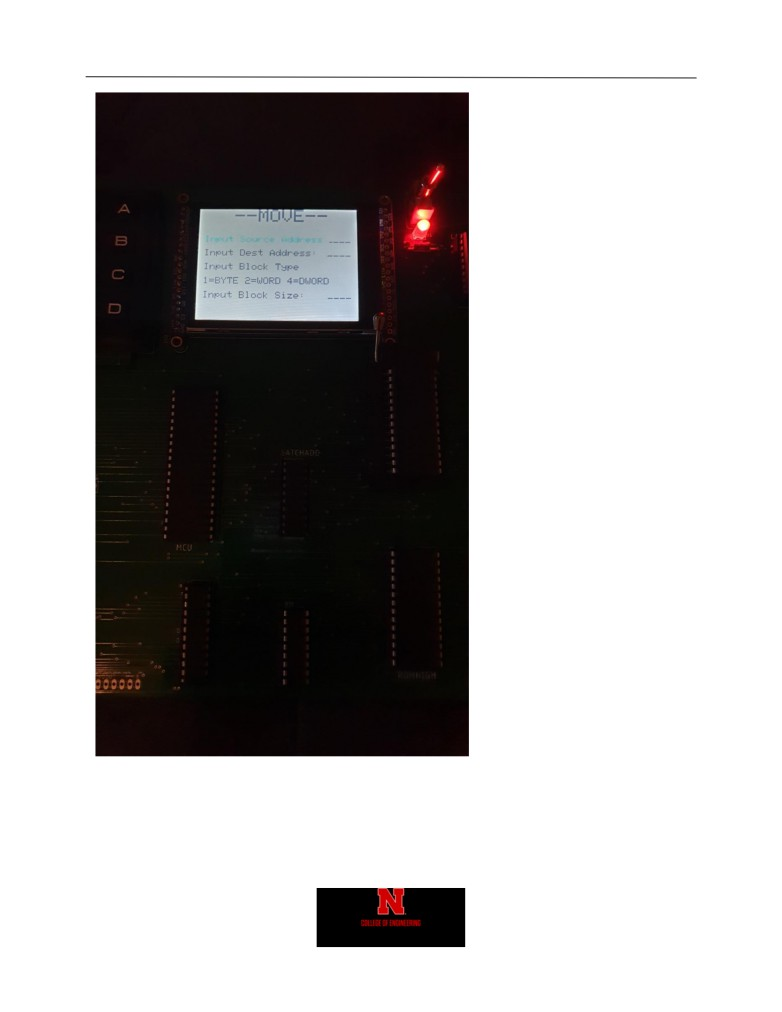Daniel Faronbi
20
Figure 11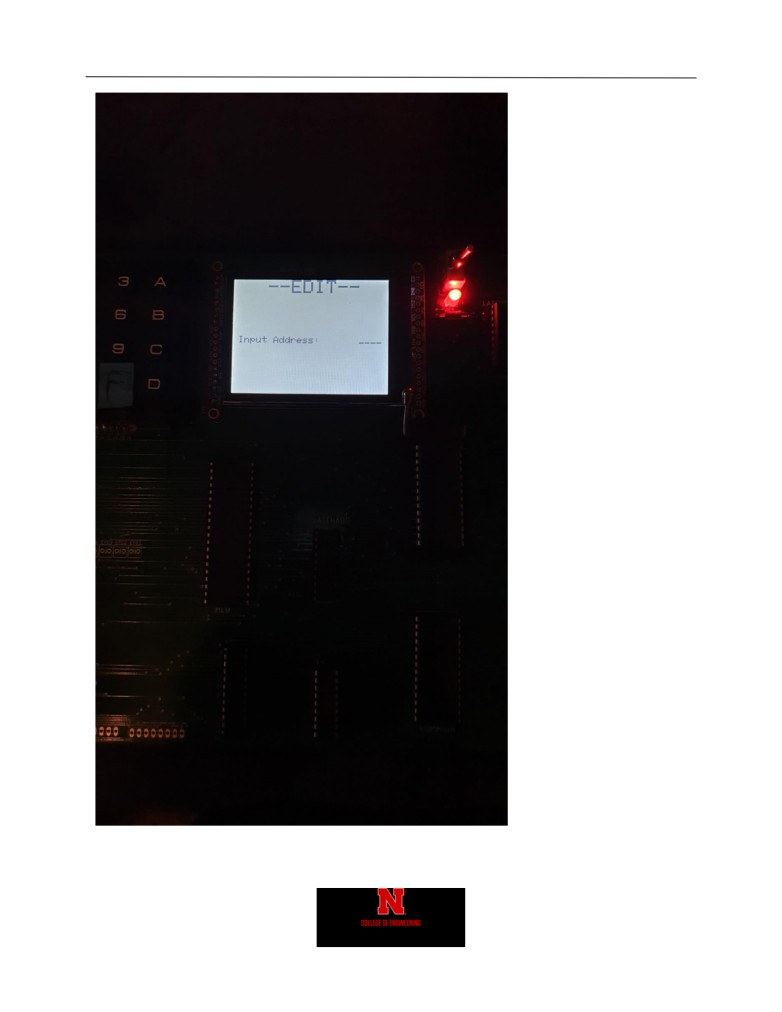Daniel Faronbi
21
Figure 12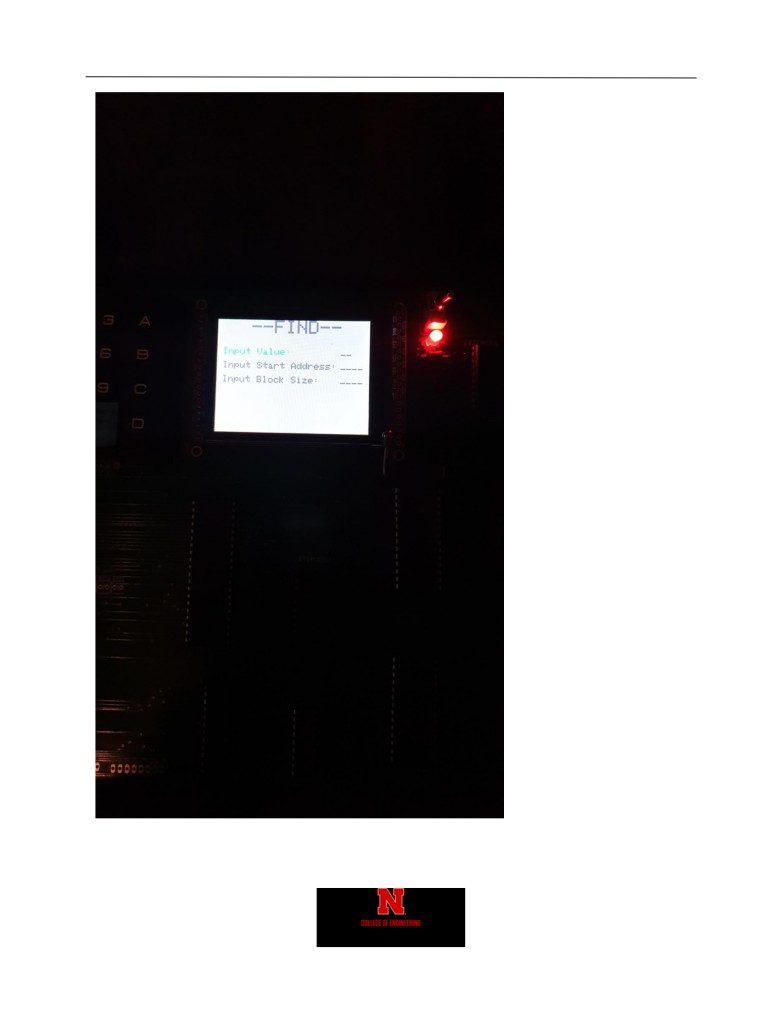Daniel Faronbi
22
Figure 13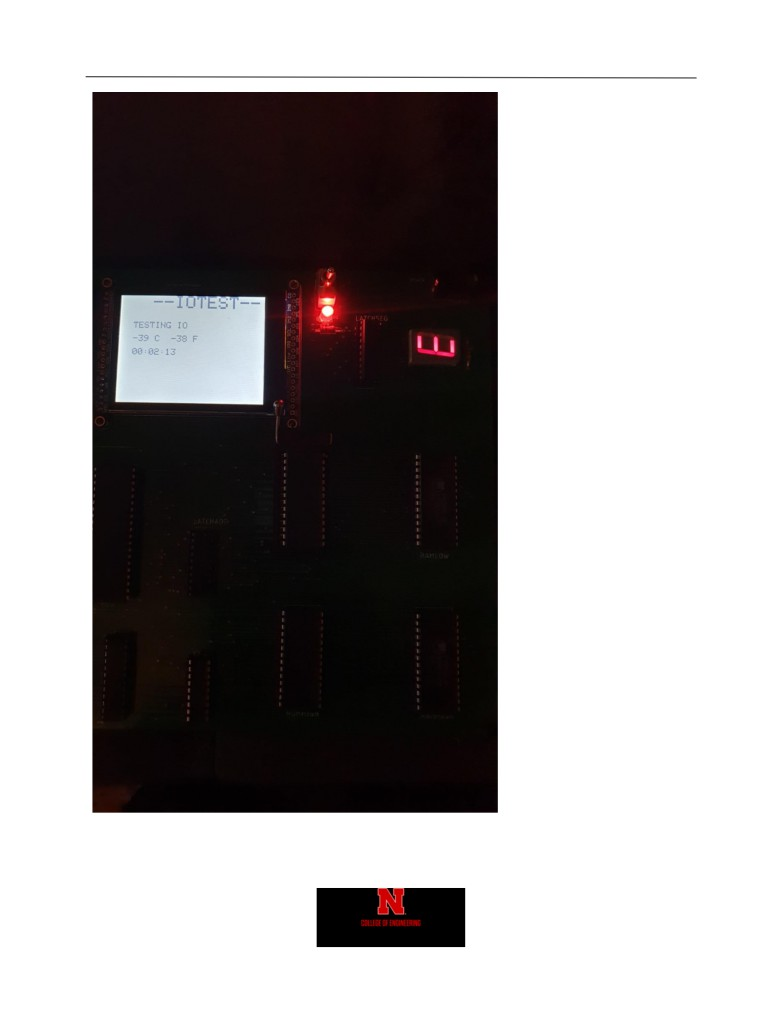Daniel Faronbi
23
Figure 14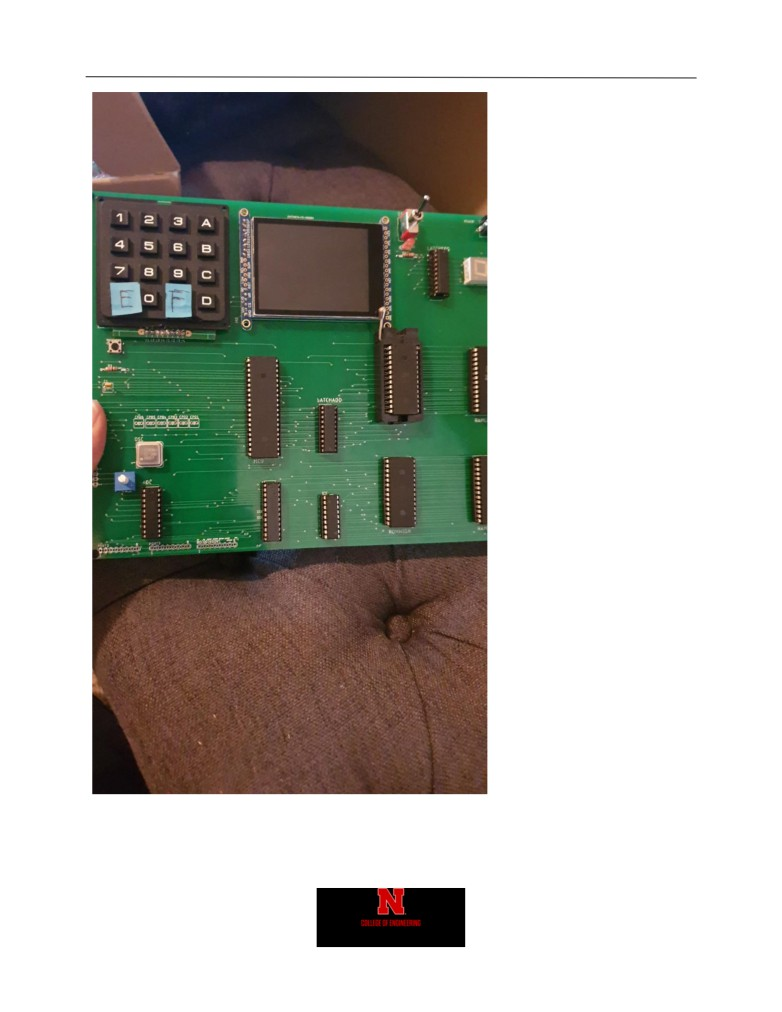Daniel Faronbi
24
Figure 15Daniel Faronbi
25
Code
/// all the required header for project here
//# include "sdcc_reg420.h"
# include <AT89C55.h>
//# include <stdio.h>
# include "registers.h"
# include "bmp_image.h"
// use a latch
# define __S1_REG__
# define __S10_REG__
# define __MI1_REG__
# define __MI10_REG__
# define __H1_REG__
# define __H10_REG__
# define __D1_REG__
# define __D10_REG__
# define __M1_REG__
# define __M10_REG__
# define __Y1_REG__
# define __Y10_REG__
# define __W_REG__
# define __REG_D__
# define __REG_E__
# define __REG_F__
// F register values
# define __HR_24__
0x04
# define __STOP__
0x02
# define __RESET__
0x01
// start RAM and end RAM
# define __START_RAM__ 0x0000
# define __END_RAM__ 0xFFFE
/// LCD specific variables
// width and height of lcd in pixels
# define TFTWIDTH 320
# define TFTHEIGHT 240
// if needed to remeber command/data lines and active/idle signals
# define __ACTIVE__ 0Daniel Faronbi
26
# define __IDLE__ 1
# define __CMD__ 0
# define __DATA__ 1
// defining important pins for LCD interfacing
// This is how it is defined for
# define IOM P3_4
# define CD P3_3
// definition of colors in 2-bytes
#define BLACK 0x0000
#define GRAY 0xD6BA
#define BLUE 0x001F
#define RED 0xF800
#define GREEN 0x07E0
#define CYAN 0x07FF
#define MAGENTA 0xF81F
#define YELLOW 0xFFE0
#define WHITE 0xFFFF
// variable definitions
#define u8 unsigned char
#define u16 unsigned int
#define u32 unsigned long
//
/// function declaration
void TFT_LCD_INIT(void); // init function
void delay(int d); // delay function for d ms
//void write8(u8 d);
//void write8Data(u8 d);
//void writeProbe(void);
void TFT_LCD_BEGIN(void); // begin LCD
void writeRegister8(u8 a, u8 d);
void writeRegister16(u16 a, u16 d);
void fillScreen(unsigned int color); // fill screen with the color defined
void setAddress(unsigned int x1,unsigned int y1,unsigned int x2,unsigned int y);
void reset(void);
// draw PIXEL at one pixel
void drawPixel(u16 x3,u16 y3,u16 colour1);
// fill your LCD in operating region
void lcdfill(u16 xsta,u16 ysta,u16 xend,u16 yend,u16 color);
void fillRect(u16 x,u16 y,u16 w,u16 h,u16 color);
// draw a characterDaniel Faronbi
27
void drawchar(int x, int y, unsigned char c,u16 color, u16 bg, u8 size);
//void write(u8 c);
// set cursor in certain pixel
void setCursor(u16 x, u16 y);
// set textcolor
void setTextColor(u16 x, u16 y);
// set textsize
void setTextSize(u8 s);
// set string write
void LCD_string_write(char *str);
// dont really need this function
void drawGrayscaleBitmap(int x, int y, const u16 bitmap[], int w, int h, u16 color) ;
// draw circles
void drawCircle(int x0, int y0, int r, u16 color);
// test circles
//void DrawRectangle(u16 x1, u16 y1, u16 x2, u16 y2);
u32 myPow(u8 m, u8 n);
void showNumberLCD(u16 x, u16 y, u32 num, u8 len);
void showNumber2LCD(u16 x, u16 y, u32 num, u8 len);
unsigned char keyDetect();
void testRAM(unsigned char d);
void freeType();
unsigned int reverse(unsigned char d);
unsigned int reverse16(unsigned int d);
void asciiToDec(unsigned char d);
void asciiToHex(unsigned char d);
inline void rtcCmd(unsigned int addr, unsigned char val);
inline void rtcWrite(unsigned int addr, unsigned char d);
void rtcInit(void);
//Memory Functions
void dump(void);
void move(void);
void edit(void);
void find(void);
//IO functions
void ioTest(void);
void rtcPrint(void);
//Extra functions
unsigned char nibbleToASCII(unsigned char key); //converts a 4 bit value to ascii
void hex4print(unsigned char num);
//print 4 bit value on LCD
void hex8print(unsigned char num);
//print 8 bit value on LCD
void hex16print(unsigned int num);
//print 16 bit value on LCD
void dec16print(unsigned int num);
//print 16 bit value as decimal on LCD
void ASCII8print(unsigned char num);
//print 8 bit ASCII value on LCD
void ASCII16print(unsigned int num);
//print 16 bit ASCII value on LCD
inline void iowrite8(unsigned char __xdata* map_address, unsigned char d);
inline void ramWrite8(unsigned char __xdata* map_address, unsigned char d);
// you have to have latch and proper decoding technique
//
*lcd_address = d; // use this in inline function along with WR signal
// to interface with address decder
// this is inline funciton definition with just a portDaniel Faronbi
28
// *** inline functions make your program runs faster
// see that I am writing directly to the port.
// you can use *lcd_address to write the data into databus
// however, you need to change decoding.
// my write probing will not work
Main C File
/*
author: Daniel Faronbi
version: v5
version is checked for:
1) removing RD pin will not guarantee working LCD (blank screen)
2) address decoding not checked yet.
3) Chip select is only used in inline function
4) changing the port worked in trainer board
5) check how much power it is drawing? if it is more than 2A?
/// The sole reason to provide this code is to make your TFTLCD (ILI9341)
/// up and running
/// the driver written for this code is for ILI9341 (check back of your LCD)
/// I don't have rotation implemented. and most of the code is in one place.
/// The only reason I have all the code in one place is because, I did not
/// want to make it more complex as it is right now.
/// for instance I will have two versions of this code. One everything at
/// one place except register definitions, another with header files.
/// Use inline assembly program as you please.
///// ======= check the defined macros =============
/// ** the code uses P0 for 8-bit interface
/// ** IOM --> P3^0
/// ** CD --> P3^1
//// connect WR and RD pin to microcontroller's RD and WR pin
////
/// ** RD --> P3^3
/// ** WR --> P3^2
/// ** RST --> P3^4
**** to quickly test your code You can use P1 and P3 for data and control signals ****
/// Also I have used mulitple inline functions for making the code significantly
/// faster. When working with them just be careful how they are implemented and why.
/// Finally, for address decoding and using data bus appropriately, you have to change
/// inline functions appropriately. There will be a version available as soon as I
/// get a working board, which on of you will be building to test my code.
/// I am sorry that I can not post this code in GITHUB right now, and I expect neither of youDaniel Faronbi
29
/// will post this code or any code written for this course in GITHUB.
*/
/// *************** IMPORTANT *********************
// It may need redfinition of pins like
// #include <reg51.h> // change microcontroller header when using AT89C55WD
# include "ecen4330lcdh.h"
# include "font.h"
//# include <string.h>
{{0x1,0x4,0x7,0xe},
{0x2,0x5,0x8,0x0},
{0x3,0x6,0x9,0xf},
{0xa,0xb,0xc,0xd} };
unsigned char colloc, rowloc;
// store it in a variable the lcd address
unsigned char pick;
# define write8inline(d) {
\
IOM = 1;
\
\
IOM = 0;
\
}
# define write8 write8inline
// data write
# define write8DataInline(d) {
\
CD = 1;
\
write8(d);
\
}
// command or register write
# define write8RegInline(d) {
\
CD = 0;
\
write8(d);
\
}
// inline definitions
# define write8Reg write8RegInline
# define write8Data write8DataInline
# define selectColor CYAN
# define backgroundColor WHITE
# define textColor GRAY
u16 cursor_x, cursor_y; /// cursor_y and cursor_x globals
u8 textsize, rotation; /// textsize and rotationDaniel Faronbi
30
u16
textcolor,
////< 16-bit background color for print()
textbgcolor;
////< 16-bit text color for print()
u16
_width,
////< Display width as modified by current rotation
_height;
////< Display height as modified by current rotation
inline void iowrite8(unsigned char __xdata* map_address, unsigned char d) {
IOM = 1;
IOM = 0;
}
unsigned char d = 0;
IOM = 1;
IOM = 0;
return d;
}
inline void ramWrite8(unsigned char __xdata* map_address, unsigned char d) {
IOM = 0;
IOM = 1;
}
unsigned char d = 0;
IOM = 0;
IOM = 1;
return d;
}
/// +=======================RTC functions===============================
void rtcInit(void){
//rtcCmd(__REG_F__, __HR_24__);
unsigned int i;
rtcCmd(__REG_F__, __HR_24__|__STOP__|__RESET__); // stop and reset
// clear the registers
for (i = __S1_REG__; i < __REG_D__;i++) {
rtcWrite(i, 0x00);
}
rtcCmd(__REG_F__, __HR_24__);
}
void rtcBusy(void){
__xdata unsigned char* map_address = (unsigned char __xdata*) (__REG_D__);
}
inline void rtcCmd(unsigned int addr, unsigned char d){
}
inline void rtcWrite(unsigned int addr, unsigned char d) {Daniel Faronbi
31
rtcCmd(__REG_D__, 0x01);
rtcBusy();
rtcCmd(__REG_D__, d);
}
unsigned char d;
rtcCmd(__REG_D__, 0x01); // hold on
rtcBusy();
d = (d & 0x0f) | 0x30; // ascii the lower word
rtcCmd(__REG_D__, 0x00); // hold off
return d;
}
void rtcPrint(void){
unsigned char mi1, mi10, s1, s10, h1, h10;
unsigned char printval;
printval = '\0'; // end with a null character for string
printval = ':';
printval = ':';
mi1 = 0x30; mi10=0x30;s1=0x30;s10=0x30;h1=0x30;h10=0x30; // char zero
printval = h10;
printval = h1;
printval = mi10;
printval = mi1;
printval = s10;
printval = s1;
setCursor(30,120);
LCD_string_write(printval);
}
// +===================================================================
void delay (int d) /// x 1ms
{
int i,j;
for (i=0;i<d;i++) /// this is For(); loop delay used to define delay value in Embedded C
{
for (j=0;j<1000;j++);
}
}
void writeRegister8(u8 a, u8 d) {
//IOM = 0;
CD = __CMD__;
write8(a);
CD = __DATA__;
write8(d);Daniel Faronbi
32
//IOM = 1;
}
void writeRegister16(u16 a, u16 d){
unsigned short int hi, lo;
hi = (a) >> 8;
lo = (a);
//IOM = 0;
//
CD = 0;
write8Reg(hi);
write8Reg(lo);
hi = (d) >> 8;
lo = (d);
CD = 1 ;
write8Data(hi);
write8Data(lo);
//
IOM =1;
}
void setCursor(u16 x, u16 y){
cursor_x = x;
cursor_y = y;
}
// set text color
void setTextColor(u16 x, u16 y){
textcolor = x;
textbgcolor = y;
}
// set text size
void setTextSize(u8 s){
if (s > 8) return;
textsize = (s>0) ? s : 1 ;
}
void setAddress(unsigned int x1,unsigned int y1,unsigned int x2,unsigned int y2){
//IOM =0;
write8Reg(0x2A);
write8Data(x1 >> 8);
write8Data(x1);
write8Data(x2 >> 8);
write8Data(x2);
write8Reg(0x2B);
write8Data(y1 >> 8);
write8Data(y1);
write8Data(y2 >> 8);
write8Data(y2);
//write8Reg(0x2C);
//IOM =1;
}
void TFT_LCD_INIT(void){
//char ID;
///int id;
_width = TFTWIDTH;
_height = TFTHEIGHT;Daniel Faronbi
33
// all low
IOM = 0;
//RDN = 1;
CD = 1;
//RST = 0;
//delay(50);
//RST = 1;
write8Reg(0x00);
write8Data(0x00);write8Data(0x00);write8Data(0x00);
//IOM = 1;
delay(200);
//IOM = 0;
writeRegister8(ILI9341_SOFTRESET, 0);
delay(50);
writeRegister8(ILI9341_DISPLAYOFF, 0);
delay(10);
writeRegister8(ILI9341_POWERCONTROL1, 0x23);
writeRegister8(ILI9341_POWERCONTROL2, 0x11);
write8Reg(ILI9341_VCOMCONTROL1);
write8Data(0x3d);
write8Data(0x30);
writeRegister8(ILI9341_VCOMCONTROL2, 0xaa);
write8Reg(ILI9341_PIXELFORMAT);
write8Data(0x55);write8Data(0x00);
writeRegister16(ILI9341_FRAMECONTROL, 0x001B);
writeRegister8(ILI9341_ENTRYMODE, 0x07);
/* writeRegister32(ILI9341_DISPLAYFUNC, 0x0A822700);*/
writeRegister8(ILI9341_SLEEPOUT, 0);
delay(150);
writeRegister8(ILI9341_DISPLAYON, 0);
delay(500);
///************* Start Initial Sequence ILI9341 controller **********///
// IOM = 1;
}
void drawPixel(u16 x3,u16 y3,u16 color1)
{
// not using to speed up
//if ((x3 < 0) ||(x3 >= TFTWIDTH) || (y3 < 0) || (y3 >= TFTHEIGHT))
//{
//
return;
//}
//IOM = 0;Daniel Faronbi
34
CD=0; write8(0x2C);
CD = 1;
write8(color1>>8);write8(color1);
//IOM = 1;
}
// draw a circle with this function
void drawCircle(int x0, int y0, int r, u16 color){
int f = 1 - r;
int ddF_x = 1;
int ddF_y = -2 * r;
int x = 0;
int y = r;
drawPixel(x0 , y0+r, color);
drawPixel(x0 , y0-r, color);
drawPixel(x0+r, y0 , color);
drawPixel(x0-r, y0 , color);
while (x<y) {
if (f >= 0) {
y--;
ddF_y += 2;
f += ddF_y;
}
x++;
ddF_x += 2;
f += ddF_x;
drawPixel(x0 + x, y0 + y, color);
drawPixel(x0 - x, y0 + y, color);
drawPixel(x0 + x, y0 - y, color);
drawPixel(x0 - x, y0 - y, color);
drawPixel(x0 + y, y0 + x, color);
drawPixel(x0 - y, y0 + x, color);
drawPixel(x0 + y, y0 - x, color);
drawPixel(x0 - y, y0 - x, color);
}
}
void fillRect(u16 x,u16 y,u16 w,u16 h,u16 color){
if ((x >= TFTWIDTH) || (y >= TFTHEIGHT))
{
return;
}
if ((x+w-1) >= TFTWIDTH)
{
w = TFTWIDTH-x;
}
if ((y+h-1) >= TFTHEIGHT)
{
h = TFTHEIGHT-y;
}
//IOM = 0;Daniel Faronbi
35
write8Reg(0x2C);
//IOM = 1; IOM = 0;
CD = 1;
for(y=h; y>0; y--)
{
for(x=w; x>0; x--)
{
write8(color>>8); write8(color);
}
}
//IOM = 1;
}
void fillScreen(unsigned int Color){
//unsigned char VH,VL;
long len = (long)TFTWIDTH * (long)TFTHEIGHT;
int blocks;
unsigned char i, hi = Color >> 8,
lo = Color;
blocks = (u16)(len / 64); // 64 pixels/block
//IOM = 0;
write8Reg(0x2C);
//IOM = 1; IOM = 0;
CD = 1;
write8(hi); write8(lo);
len--;
while(blocks--) {
i = 16; // 64 pixels/block / 4 pixels/pass
do {
write8(hi); write8(lo);write8(hi); write8(lo);
write8(hi); write8(lo);write8(hi); write8(lo);
} while(--i);
}
for(i = (char)len & 63; i--; ) {
write8(hi); write8(lo);
}
//IOM = 1;
}
void drawChar(int x, int y, unsigned char c,u16 color, u16 bg, u8 size){
if ((x >=TFTWIDTH) || // Clip right
(y >=TFTHEIGHT)
|| // Clip bottom
((x + 6 * size - 1) < 0) || // Clip left
((y + 8 * size - 1) < 0))
// Clip top
{
return;
}Daniel Faronbi
36
for (char i=0; i<6; i++ )
{
u8 line;
if (i == 5)
{
line = 0x0;
}
else
{
}
for (char j = 0; j<8; j++)
{
if (line & 0x1)
{
if (size == 1) // default size
{
drawPixel(x+i, y+j, color);
}
else { // big size
fillRect(x+(i*size), y+(j*size), size, size, color);
}
} else if (bg != color)
{
if (size == 1) // default size
{
drawPixel(x+i, y+j, bg);
}
else
{
// big size
fillRect(x+i*size, y+j*size, size, size, bg);
}
}
line >>= 1;
}
}
}
void write(u8 c)//write a character at setted coordinates after setting location and colour
{
if (c == '\n')
{
cursor_y += textsize*8;
cursor_x = 0;
}
else if (c == '\r')
{
// skip em
}
else
{
drawChar(cursor_x, cursor_y, c, textcolor, textbgcolor, textsize);
cursor_x += textsize*6;
}
}
void LCD_string_write(char *str)
{
int i;
for(i=0;str[i]!=0;i++)
/* Send each char of string till the NULL */Daniel Faronbi
37
{
write(str[i]);
/* Call transmit data function */
}
}
// test RAM function
void testRAM(unsigned char d){
unsigned int i;
for (i = __START_RAM__; i<__END_RAM__; i++) {
IOM = 0;
IOM = 1;
}
}
void freeType() {
unsigned char count = 0;
unsigned char d;
while(1){
if (count == 8) {
d = '\n';
count = 0;
write(d);
}
else{
d = keyDetect();
write(d);
}
count++;
}
}
unsigned char keyDetect()
{
/*set port direction as input-output*/
do
{
colloc&= 0xF0;
}while(colloc != 0xF0);
/* read status of column */
do
{
do
{
delay(20); /* 20ms key debounce time */
/* read status of column */
}while(colloc == 0xF0);
/* check for any key press */
delay(1);
}while(colloc == 0xF0);Daniel Faronbi
38
while(1)
{
/* now check for rows */
/* check for pressed key in 1st row */
if(colloc != 0xF0)
{
rowloc = 0;
break;
}
/* check for pressed key in 2nd row */
if(colloc != 0xF0)
{
rowloc = 1;
break;
}
/* check for pressed key in 3rd row */
if(colloc != 0xF0)
{
rowloc = 2;
break;
}
/* check for pressed key in 4th row */
if(colloc != 0xF0)
{
rowloc = 3;
break;
}
}
if(colloc == 0xE0)
{
}
else if(colloc == 0xD0)
{
}
else if(colloc == 0xB0)
{
}
else
{
}
}
unsigned int reverse(unsigned char d) {
unsigned int rev = 0;
unsigned int val = 0;
while(d >= 1){
val = d%10;
d = d/10;Daniel Faronbi
39
rev = rev * 10 + val;
}
return rev;
}
unsigned int reverse16(unsigned int d) {
unsigned int rev = 0;
unsigned int val = 0;
while(d >= 1){
val = d%10;
d = d/10;
rev = rev * 10 + val;
}
return rev;
}
void asciiToDec(unsigned char d) {
unsigned char val;
unsigned int id;
id = reverse(d);
while (id >= 1){
val = id % 10;
id = id/10;
write(val + '0');
}
write('\n');
}
void asciiToHex(unsigned char d) {
unsigned char val;
unsigned char store;
unsigned char i =0;
store = 0;
store = 0;
while (d >= 1){
val = d % 16;
d = d/16;
if (val <= 9) {
store[i] = val + '0';
}
else {
store[i] = (val%10) + 'A';
}
i++;
}
write(store);
write(store);
//write('\n');
}
void writeSomeLines(){
fillScreen(BLACK);
setTextColor(RED, BLACK);
setTextSize(5);
LCD_string_write("Welcome\n");
setTextColor(RED, BLACK);
setTextSize(3);
LCD_string_write("ECEN-4330\n");
setCursor(0,210);
setTextSize(2);
LCD_string_write("Daniel Faronbi\n");Daniel Faronbi
40
delay(100);
}
unsigned char d;
float cel;
float far;
setCursor(30, 90);
LCD_string_write("
");
IOM = 1;
cel = (((((float)d)+1.0)/256.0) * 165.0)-40.0;
far = (cel*(9.0/5.0))+32.0;
setCursor(30, 90);
if(cel >=0){
dec16print((int)cel);
LCD_string_write(" C ");
dec16print((int)far);
LCD_string_write(" F ");
}
if(cel < 0){
LCD_string_write("-");
dec16print(((int)cel)*-1);
LCD_string_write(" C ");
LCD_string_write("-");
dec16print(((int)far)*-1);
LCD_string_write(" F ");
}
delay(50);
}
fillScreen(backgroundColor);
setTextSize(4);
setTextColor(textColor, backgroundColor);
setCursor(0, 0);
LCD_string_write("
setTextSize(3);
setCursor(0, 60);
LCD_string_write(" D: DUMP\n");
setCursor(170, 60);
LCD_string_write(" B: MOVE\n");
setCursor(0, 120);
LCD_string_write(" E: EDIT\n");
setCursor(170, 120);
LCD_string_write(" F: FIND\n");
setCursor(0, 180);
LCD_string_write(" A: IO TEST\n");
setTextColor(selectColor, backgroundColor);
}
void dump(void){
unsigned char __xdata* d;
__idata unsigned int startAdd = 0;
__idata unsigned int blockSize = 0;
__idata unsigned int page = 0;
__idata unsigned char in;Daniel Faronbi
41
__idata unsigned char blockType =0;
__idata unsigned char exit =1;
fillScreen(backgroundColor);
setTextSize(4);
setTextColor(textColor, backgroundColor);
setCursor(0, 0);
LCD_string_write("
--DUMP--\n");
setTextSize(2);
setCursor(0, 60);
setCursor(260, 60);
LCD_string_write("____");
setCursor(0, 90);
LCD_string_write(" Input Block Type");
setCursor(0, 120);
LCD_string_write(" 1=BYTE 2=WORD 4=DWORD");
setCursor(0, 150);
LCD_string_write(" Input Block Size:");
setCursor(260, 150);
LCD_string_write("____");
setTextColor(selectColor, backgroundColor);
setCursor(0, 60);
setCursor(260, 60);
in = keyDetect();
hex4print(in);
startAdd += in * 16 * 16 * 16; //put in 4th hexidecimal place
in = keyDetect();
hex4print(in);
startAdd += in * 16 * 16; //put in 3rd hexidecimal place
in = keyDetect();
hex4print(in);
startAdd += in * 16; //put in 2nd hexidecimal place
in = keyDetect();
hex4print(in);
startAdd += in ; //put in 1st hexidecimal place
setTextColor(textColor, backgroundColor);
setCursor(0, 60);
setTextColor(selectColor, backgroundColor);
setCursor(0, 90);
LCD_string_write(" Input Block Type");
while(blockType != 1 && blockType != 2 && blockType != 4)
{
blockType = keyDetect();
if(blockType == 1){
setCursor(0, 120);
setTextColor(selectColor, backgroundColor);
LCD_string_write(" 1=BYTE ");
setTextColor(textColor, backgroundColor);
LCD_string_write("2=WORD 4=DWORD");
delay(20);
}Daniel Faronbi
42
if(blockType == 2){
setCursor(0, 120);
setTextColor(textColor, backgroundColor);
LCD_string_write(" 1=BYTE ");
setTextColor(selectColor, backgroundColor);
LCD_string_write("2=WORD ");
setTextColor(textColor, backgroundColor);
LCD_string_write("4=DWORD");
delay(20);
}
if(blockType == 4){
setCursor(0, 120);
setTextColor(textColor, backgroundColor);
LCD_string_write(" 1=BYTE 2=WORD ");
setTextColor(selectColor, backgroundColor);
LCD_string_write("4=DWORD");
delay(20);
}
}
setTextColor(textColor, backgroundColor);
setCursor(0, 90);
LCD_string_write(" Input Block Type");
setCursor(0, 150);
setTextColor(selectColor, backgroundColor);
LCD_string_write(" Input Block Size:");
setCursor(260, 150);
in = keyDetect();
hex4print(in);
blockSize += in * 16 * 16 * 16; //put in 4th hexidecimal place
in = keyDetect();
hex4print(in);
blockSize += in * 16 * 16; //put in 3rd hexidecimal place
in = keyDetect();
hex4print(in);
blockSize += in * 16; //put in 2nd hexidecimal place
in = keyDetect();
hex4print(in);
blockSize += in ; //put in 1st hexidecimal place
LCD_string_write("\n");
fillScreen(backgroundColor);
setTextSize(4);
setTextColor(textColor, backgroundColor);
setCursor(0, 0);
LCD_string_write("
--DUMP--\n");
while(exit){
setTextSize(2);
setTextColor(textColor, backgroundColor);
setCursor(0, 60);
LCD_string_write(" Page:");
setCursor(150, 60);
hex16print(page);
setCursor(0, 90);
setCursor(150, 90);Daniel Faronbi
43
setCursor(0, 120);
LCD_string_write(" Hex Data:");
setCursor(150, 120);
d = (unsigned char __xdata*)(startAdd + page * blockType);
if(blockType == 1)
{
setCursor(0, 120);
LCD_string_write(" Hex Data:");
setCursor(150, 120);
setCursor(0, 150);
LCD_string_write(" ASCII Data:");
setCursor(150, 150);
}
if(blockType == 2)
{
setCursor(0, 120);
LCD_string_write(" Hex Data:");
setCursor(150, 120);
d++;
d--;
setCursor(0, 150);
LCD_string_write(" ASCII Data:");
setCursor(150, 150);
d++;
}
if(blockType == 4)
{
setCursor(0, 120);
LCD_string_write(" Hex Data:");
setCursor(150, 120);
d++;
d++;
d++;
d--;
d--;
d--;
setCursor(0, 150);
LCD_string_write(" ASCII Data:");
setCursor(150, 150);
d++;Daniel Faronbi
44
d++;
d++;
}
setCursor(0, 180);
LCD_string_write(" 0=Next 1=Prev 2=Exit");
in = keyDetect();
if(in==0){
if(page < blockSize -1)
page++;
setCursor(0, 180);
setTextColor(selectColor, backgroundColor);
LCD_string_write(" 0=Next ");
setTextColor(textColor, backgroundColor);
LCD_string_write("1=Prev 2=Exit");
delay(20);
}
if(in==1){
if(page > 0)
page--;
setCursor(0, 180);
setTextColor(textColor, backgroundColor);
LCD_string_write(" 0=Next ");
setTextColor(selectColor, backgroundColor);
LCD_string_write("1=Prev ");
setTextColor(textColor, backgroundColor);
LCD_string_write("2=Exit");
delay(20);
}
if(in==2){
exit=0;
setCursor(0, 180);
setTextColor(textColor, backgroundColor);
LCD_string_write(" 0=Next 1=Prev ");
setTextColor(selectColor, backgroundColor);
LCD_string_write("2=Exit");
delay(20);
}
}
}
void move(void){
unsigned char __xdata* d;
__idata unsigned int destAdd = 0;
__idata unsigned int blockSize = 0;
__idata unsigned char in;
__idata unsigned char blockType =0;
fillScreen(backgroundColor);
setTextSize(4);
setTextColor(textColor, backgroundColor);
setCursor(0, 0);
LCD_string_write("
--MOVE--\n");Daniel Faronbi
45
setTextSize(2);
setCursor(0, 60);
setCursor(260, 60);
LCD_string_write("____");
setCursor(0, 90);
setCursor(260, 90);
LCD_string_write("____");
setCursor(0, 120);
LCD_string_write(" Input Block Type");
setCursor(0, 150);
LCD_string_write(" 1=BYTE 2=WORD 4=DWORD");
setCursor(0, 180);
LCD_string_write(" Input Block Size:");
setCursor(260, 180);
LCD_string_write("____");
setTextColor(selectColor,backgroundColor);
setCursor(0, 60);
setCursor(260, 60);
in = keyDetect();
hex4print(in);
sourceAdd += in * 16 * 16 * 16; //put in 4th hexidecimal place
in = keyDetect();
hex4print(in);
sourceAdd += in * 16 * 16; //put in 3rd hexidecimal place
in = keyDetect();
hex4print(in);
sourceAdd += in * 16; //put in 2nd hexidecimal place
in = keyDetect();
hex4print(in);
sourceAdd += in ; //put in 1st hexidecimal place
setTextColor(textColor,backgroundColor);
setCursor(0, 60);
setTextColor(selectColor,backgroundColor);
setCursor(0, 90);
setCursor(260, 90);
in = keyDetect();
hex4print(in);
destAdd += in * 16 * 16 * 16; //put in 4th hexidecimal place
in = keyDetect();
hex4print(in);
destAdd += in * 16 * 16; //put in 3rd hexidecimal place
in = keyDetect();
hex4print(in);
destAdd += in * 16; //put in 2nd hexidecimal place
in = keyDetect();
hex4print(in);
destAdd += in ; //put in 1st hexidecimal place
setTextColor(textColor,backgroundColor);
setCursor(0, 90);
setTextColor(selectColor,backgroundColor);Daniel Faronbi
46
setCursor(0, 120);
LCD_string_write(" Input Block Type");
while(blockType != 1 && blockType != 2 && blockType != 4)
{
blockType = keyDetect();
if(blockType == 1){
setCursor(0, 150);
setTextColor(selectColor, backgroundColor);
LCD_string_write(" 1=BYTE ");
setTextColor(textColor, backgroundColor);
LCD_string_write("2=WORD 4=DWORD");
delay(20);
}
if(blockType == 2){
setCursor(0, 150);
setTextColor(textColor, backgroundColor);
LCD_string_write(" 1=BYTE ");
setTextColor(selectColor, backgroundColor);
LCD_string_write("2=WORD ");
setTextColor(textColor, backgroundColor);
LCD_string_write("4=DWORD");
delay(20);
}
if(blockType == 4){
setCursor(0, 150);
setTextColor(textColor, backgroundColor);
LCD_string_write(" 1=BYTE 2=WORD ");
setTextColor(selectColor, backgroundColor);
LCD_string_write("4=DWORD");
delay(20);
}
}
setTextColor(textColor,backgroundColor);
setCursor(0, 120);
LCD_string_write(" Input Block Type");
setTextColor(selectColor,backgroundColor);
setCursor(0, 180);
LCD_string_write(" Input Block Size:");
setCursor(260, 180);
in = keyDetect();
hex4print(in);
blockSize += in * 16 * 16 * 16; //put in 4th hexidecimal place
in = keyDetect();
hex4print(in);
blockSize += in * 16 * 16; //put in 3rd hexidecimal place
in = keyDetect();
hex4print(in);
blockSize += in * 16; //put in 2nd hexidecimal place
in = keyDetect();
hex4print(in);
blockSize += in ; //put in 1st hexidecimal placeDaniel Faronbi
47
}
setTextColor(textColor,backgroundColor);
setCursor(0, 210);
LCD_string_write("Done!!!");
delay(60);
}
void edit(void){
unsigned char __xdata* d;
__idata unsigned int address = 0;
__idata unsigned char in;
__idata unsigned char value = 0;
__idata unsigned char exit =1;
fillScreen(backgroundColor);
setTextSize(4);
setTextColor(textColor, backgroundColor);
setCursor(0, 0);
LCD_string_write("
--EDIT--\n");
setTextSize(2);
setCursor(0, 120);
setCursor(260, 120);
LCD_string_write("____");
setTextColor(selectColor, backgroundColor);
setCursor(260, 120);
in = keyDetect();
hex4print(in);
address += in * 16 * 16 * 16; //put in 4th hexidecimal place
in = keyDetect();
hex4print(in);
address += in * 16 * 16; //put in 3rd hexidecimal place
in = keyDetect();
hex4print(in);
address += in * 16; //put in 2nd hexidecimal place
in = keyDetect();
hex4print(in);
address += in ; //put in 1st hexidecimal place
fillScreen(backgroundColor);
setTextSize(4);
setTextColor(textColor, backgroundColor);
setCursor(0, 0);
LCD_string_write("
--EDIT--\n");
while(exit){
setTextSize(2);
setCursor(0, 60);
setCursor(260, 60);
setCursor(0, 90);
LCD_string_write("Content:");
setCursor(260, 90);Daniel Faronbi
48
setCursor(0, 120);
LCD_string_write("New Value:");
setCursor(260, 120);
LCD_string_write("__");
setCursor(0, 150);
LCD_string_write("Choose Next Action");
setCursor(0, 180);
LCD_string_write(" 0=NEXT 1=EXIT");
setTextColor(selectColor,backgroundColor);
setCursor(0, 120);
LCD_string_write("New Value:");
setCursor(260, 120);
in = keyDetect();
hex4print(in);
value += in * 16; //put in 2nd hexidecimal place
in = keyDetect();
hex4print(in);
value += in ; //put in 1st hexidecimal place
ramWrite8(d,value);
//write new value to memory
value = 0;
setTextColor(textColor,backgroundColor);
setCursor(0, 120);
LCD_string_write("New Value:");
setTextColor(selectColor,backgroundColor);
setCursor(0, 150);
LCD_string_write("Choose Next Action");
in = keyDetect();
if(in==0){
setTextColor(selectColor,backgroundColor);
setCursor(0, 180);
LCD_string_write(" 0=NEXT ");
setTextColor(textColor,backgroundColor);
LCD_string_write("1=EXIT");
delay(20);
}
if(in==1){
exit=0;
setTextColor(textColor,backgroundColor);
setCursor(0, 180);
LCD_string_write(" 0=NEXT ");
setTextColor(selectColor,backgroundColor);
LCD_string_write("1=EXIT");
delay(20);
}
setTextColor(textColor,backgroundColor);
setCursor(0, 150);
LCD_string_write("Choose Next Action");
setCursor(0, 180);
LCD_string_write(" 0=NEXT 1=EXIT");
}
}
void find(void){
unsigned char __xdata* d;Daniel Faronbi
49
__idata unsigned int startAdd = 0;
__idata unsigned int blockSize = 0;
__idata unsigned char in;
__idata unsigned char value = 0;
__idata unsigned char noneFound = 1;
fillScreen(backgroundColor);
setTextSize(4);
setTextColor(textColor, backgroundColor);
setCursor(0, 0);
LCD_string_write("
--FIND--\n");
setTextSize(2);
setCursor(0, 60);
LCD_string_write(" Input Value:");
setCursor(260, 60);
LCD_string_write("__");
setCursor(0, 90);
setCursor(260, 90);
LCD_string_write("____");
setCursor(0, 120);
LCD_string_write(" Input Block Size:");
setCursor(260, 120);
LCD_string_write("____");
setTextColor(selectColor, backgroundColor);
setCursor(0, 60);
LCD_string_write(" Input Value:");
setCursor(260, 60);
in = keyDetect();
hex4print(in);
value += in * 16; //put in 2nd hexidecimal place
in = keyDetect();
hex4print(in);
value += in ; //put in 1st hexidecimal place
setTextColor(textColor, backgroundColor);
setCursor(0, 60);
LCD_string_write(" Input Value:");
setTextColor(selectColor, backgroundColor);
setCursor(0, 90);
setCursor(260, 90);
in = keyDetect();
hex4print(in);
startAdd += in * 16 * 16 * 16; //put in 4th hexidecimal place
in = keyDetect();
hex4print(in);
startAdd += in * 16 * 16; //put in 3rd hexidecimal place
in = keyDetect();
hex4print(in);
startAdd += in * 16; //put in 2nd hexidecimal place
in = keyDetect();
hex4print(in);
startAdd += in ; //put in 1st hexidecimal place
setTextColor(textColor, backgroundColor);
setCursor(0, 90);Daniel Faronbi
50
setTextColor(selectColor, backgroundColor);
setCursor(0, 120);
LCD_string_write(" Input Block Size:");
setCursor(260, 120);
in = keyDetect();
hex4print(in);
blockSize += in * 16 * 16 * 16; //put in 4th hexidecimal place
in = keyDetect();
hex4print(in);
blockSize += in * 16 * 16; //put in 3rd hexidecimal place
in = keyDetect();
hex4print(in);
blockSize += in * 16; //put in 2nd hexidecimal place
in = keyDetect();
hex4print(in);
blockSize += in ; //put in 1st hexidecimal place
fillScreen(backgroundColor);
setTextSize(4);
setTextColor(textColor, backgroundColor);
setCursor(0, 0);
LCD_string_write("
--FIND--\n");
setTextSize(2);
setCursor(0, 70);
LCD_string_write(" ______________");
setCursor(0, 60);
LCD_string_write(" Value Found At");
for(unsigned int i = 0; i < blockSize; i++){
setCursor(60, 120);
noneFound = 0;
setTextColor(textColor, backgroundColor);
setCursor(0, 180);
LCD_string_write(" 0=Next 1=Exit");
in = keyDetect();
if(in == 0){
setTextColor(selectColor, backgroundColor);
setCursor(0, 180);
LCD_string_write(" 0=Next ");
setTextColor(textColor, backgroundColor);
LCD_string_write("1=Exit");
delay(20);
}
if(in == 1){
setTextColor(textColor, backgroundColor);
setCursor(0, 180);
LCD_string_write(" 0=Next ");
setTextColor(selectColor, backgroundColor);
LCD_string_write("1=Exit");
delay(20);
break;
}
}
}Daniel Faronbi
51
if(noneFound){
setTextColor(textColor, backgroundColor);
setCursor(0, 180);
LCD_string_write(" NONE FOUND!!!");
}
delay(50);
}
void ioTest(void){
fillScreen(backgroundColor);
setTextSize(4);
setTextColor(textColor, backgroundColor);
setCursor(0, 0);
LCD_string_write("
--IOTEST--\n");
setTextSize(2);
setCursor(30, 60);
LCD_string_write("TESTING IO");
for(unsigned int i = 0; i<65535; i++)
rtcPrint();
for(unsigned int i = 0; i<65535; i++)
rtcPrint();
for(unsigned int i = 0; i<65535; i++)
rtcPrint();
for(unsigned int i = 0; i<65535; i++)
rtcPrint();
for(unsigned int i = 0; i<65535; i++)
rtcPrint();
for(unsigned int i = 0; i<65535; i++)
rtcPrint();
for(unsigned int i = 0; i<65535; i++)Daniel Faronbi
52
rtcPrint();
for(unsigned int i = 0; i<65535; i++)
rtcPrint();
for(unsigned int i = 0; i<65535; i++)
rtcPrint();
for(unsigned int i = 0; i<65535; i++)
rtcPrint();
for(unsigned int i = 0; i<65535; i++)
rtcPrint();
for(unsigned int i = 0; i<65535; i++)
rtcPrint();
for(unsigned int i = 0; i<65535; i++)
rtcPrint();
for(unsigned int i = 0; i<65535; i++)
rtcPrint();
for(unsigned int i = 0; i<65535; i++)
rtcPrint();
for(unsigned int i = 0; i<65535; i++)
rtcPrint();
setCursor(0, 240);
LCD_string_write("DONE!!!");
delay(50);
}
unsigned char nibbleToASCII(unsigned char key){Daniel Faronbi
53
if(key == 0x0)
return '0';
if(key == 0x1)
return '1';
if(key == 0x2)
return '2';
if(key == 0x3)
return '3';
if(key == 0x4)
return '4';
if(key == 0x5)
return '5';
if(key == 0x6)
return '6';
if(key == 0x7)
return '7';
if(key == 0x8)
return '8';
if(key == 0x9)
return '9';
if(key == 0xa)
return 'A';
if(key == 0xb)
return 'B';
if(key == 0xc)
return 'C';
if(key == 0xd)
return 'D';
if(key == 0xe)
return 'E';
if(key == 0xf)
return 'F';
else
return 0xff;
}
void hex4print(unsigned char num){
write((u8) nibbleToASCII(num));
}
void hex8print(unsigned char num){
hex4print(num >> 4);
hex4print(num & 0x0F);
}
void hex16print(unsigned int num){
hex8print((unsigned char)(num >> 8));
hex8print((unsigned char)num);
}
void dec16print(unsigned int num){
unsigned int val;
unsigned int id;
id = reverse16(num);
while (id >= 1){
val = id % 10;
id = id/10;
write(val + '0');
}
}
void ASCII8print(unsigned char num){Daniel Faronbi
54
write((u8)num);
}
void ASCII16print(unsigned int num){
ASCII8print((unsigned char)(num >> 8));
ASCII8print((unsigned char)num);
}
void main (void) {
TFT_LCD_INIT();
//fillScreen(CYAN);
writeSomeLines();
fillScreen(BLACK);
setTextColor(RED, BLACK);
setTextSize(2);
setCursor(0,0);
testRAM(0xAA);
for (unsigned int i = __START_RAM__; i<__END_RAM__; i++) {
IOM = 0;
if(0xAA != *(unsigned char __xdata*)(i)){
setCursor(0,0);
LCD_string_write("ERROR FOUND At: ");
hex16print(i);
delay(50);
}
IOM = 1;
}
rtcInit();
while(1) {
pick = nibbleToASCII(keyDetect());
if(pick == 'D'){
setCursor(0, 60);
LCD_string_write(" D: DUMP\n");
delay(40);
dump();
}
if(pick == 'B'){
setCursor(170, 60);
LCD_string_write(" B: MOVE\n");
delay(40);
move();
}
if(pick == 'E'){
setCursor(0, 120);
LCD_string_write(" E: EDIT\n");
delay(40);
edit();
}
if(pick == 'F'){
setCursor(170, 120);
LCD_string_write(" F: FIND\n");
delay(40);
find();Daniel Faronbi
55
}
if(pick == 'A'){
setCursor(0, 180);
LCD_string_write(" A: IO TEST\n");
delay(40);
ioTest();
}
// run your program for a while
//freeType();
}
}